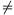Mathematical and Physical Journal
for High Schools
Issued by the MATFUND Foundation
 Already signed up? New to KöMaL?

# KöMaL Problems in Mathematics, May 2012

Show/hide problems of signs:## Problems with sign 'C'

Deadline expired on June 11, 2012.

C. 1125. A table of three columns is filled in with numbers as follows: The number 2n, without its digit in the units' place, is entered in the field at the intersection of the first column and the nth row. The digit that was cancelled in the first column is entered in the field in the nth row of the second column. Finally, the entry in the third column and the nth row will be the product of the first two entries of the same row. Prove that the numbers in the third column are all divisible by six.

(5 pont)

solution (in Hungarian), statistics

C. 1126. AB is a diameter of a circle of radius 30 cm centred at O1. This circle is touched from the inside by two other circles: at point A by a circle of radius 15 cm centred at O2, and at point B by a circle of radius 10 cm centred at O3. What is the radius of the circles that touch all three of these circles?

(5 pont)

solution (in Hungarian), statistics

C. 1127. Solve the following equation on the set of real numbers: |x-|x-|x-4|||=x2-4x.

(5 pont)

solution (in Hungarian), statistics

C. 1128. Six dice are rolled simultaneously. What is the probability that at least four identical numbers are rolled?

(5 pont)

solution (in Hungarian), statistics

C. 1129. The tangents drawn from a certain point to the parabola 2y=x2-2 are also tangent to the parabola 4y=x2-10x+37. Find the point.

Suggested by G. Holló, Budapest

(5 pont)

solution (in Hungarian), statistics## Problems with sign 'B'

Deadline expired on June 11, 2012.

B. 4452. Let t>0 be a real number, and let Ti denote the sum(for all positive integers i). Prove thatfor all positive integers k.

Suggested by J. Pataki, Budapest

(3 pont)

solution (in Hungarian), statistics

B. 4453. With three planes, a cuboid T is divided into eight smaller cuboids. The small cuboids are coloured black and white such that adjacent pieces have different colours. Given that the sum of the volumes of the white cuboids equals the sum of the volumes of the black cuboids, show that one of the planes halves the volume of T.

(4 pont)

solution (in Hungarian), statistics

B. 4454. In a parallelogram ABCD, AB>BC. Construct that point P in the interior of the parallelogram for whichAPD+BPC=180o andPAB+PDA=90o.

(4 pont)

solution (in Hungarian), statistics

B. 4455. Consider a finite set of positive numbers such that no number is greater than the sum of the other numbers. Prove that it is possible to partition the numbers into two sets such that the sum of the numbers in one set is at most twice the sum of the numbers in the other set.

A problem by Paul Erdős, 1913-1996

(3 pont)

solution (in Hungarian), statistics

B. 4456. Let f be a real function defined on the set of positive real numbers such thatfor all x,y>0. Prove that f is a constant function.

Suggested by Z. Daróczy, Debrecen

(3 pont)

solution (in Hungarian), statistics

B. 4457. How long is the shortest line segment that halves the area of the triangle with sides 3, 4 and 5?

(4 pont)

solution (in Hungarian), statistics

B. 4458. Lines a and b are given lines in the plane, A is a given point not lying on a, and B is a given point not lying on b. If point O does not coincide with A or B, then there exists a transformation composed of a rotation about O and a central enlargement from O, such that A is mapped to B. What is the locus of those points in the plane for which this transformation maps the intersection of lines OA and a to the intersection of lines OB and b?

(4 pont)

solution (in Hungarian), statistics

B. 4459. For x>1, let A(x) denote the sum of reciprocals of the positive square-free numbers less than x, and let B(x) denote the sum of reciprocals of the non-square-free positive numbers less than x. Prove that A(x)>B(x).

Suggested by P. Maga)

(4 pont)

solution (in Hungarian), statistics

B. 4460. Regular triangles ABD, BCE and CAF are drawn over the sides of a triangle ABC, on the outside. Let G and H denote the midpoints of the line segments BD and BE, respectively, and let the midpoint of triangle CAF be I. Prove that the lines AH, CG and BI are concurrent.

Suggested by Sz. Miklós, Herceghalom

(5 pont)

solution (in Hungarian), statistics

B. 4461. Let p2 be a real number. Prove that (x+y)p+(z+v)p+(x+z)p+(y+v)pxp+yp+zp+vp+(x+y+z+v)p for all non-negative real numbers x, y, z and v.

Suggested by Á. Besenyei, Budapest

(5 pont)

solution (in Hungarian), statistics## Problems with sign 'A'

Deadline expired on June 11, 2012.

A. 563. Let 1p<2 be a real number. Prove that (x+y)p+(z+v)p+(x+z)p+(y+v)pxp+yp+zp+vp+(x+y+z+v)p for all nonnegative real numbers x, y, z and v.

Proposed by Ádám Besenyei, Budapest

(5 pont)

solution (in Hungarian), statistics

A. 564. Let k be the incircle in the triangle ABC, which is tangent to the sides AB, BC, CA at the points C0, A0 and B0, respectively. The angle bisector starting at A meets k at A1 and A2, the angle bisector starting at B meets k at B1 and B2; AA1<AA2 and BB1<BB2. The circle k1k is tangent externally to the side CA at B0 and it is tangent to the line AB. The circle k2k is tangent externally to the side BC at A0, and it is tangent to the line AB. The circle k3 is tangent to k at A1, and it is tangent to k1 at point P. The circle k4 is tangent to k at B1, and it is tangent to k2 at point Q. Prove that the radical axis between the circles A1A2P and B1B2Q is the angle bisector starting at C.

(5 pont)

statistics

A. 565. The positive integers are coloured with a finite number of colours. A function f from the set of positive integers to itself has the following two properties:

(a) if xy, then f(x)f(y); and

(b) if x, y, and z are (not necessarily distinct) positive integers of the same colour and x+y=z, then f(x)+f(y)=f(z).

Does it follow that the functionis bounded from above?

(Based on Romanian Master in Mathematics, problem 2012/3)

(5 pont)

statistics